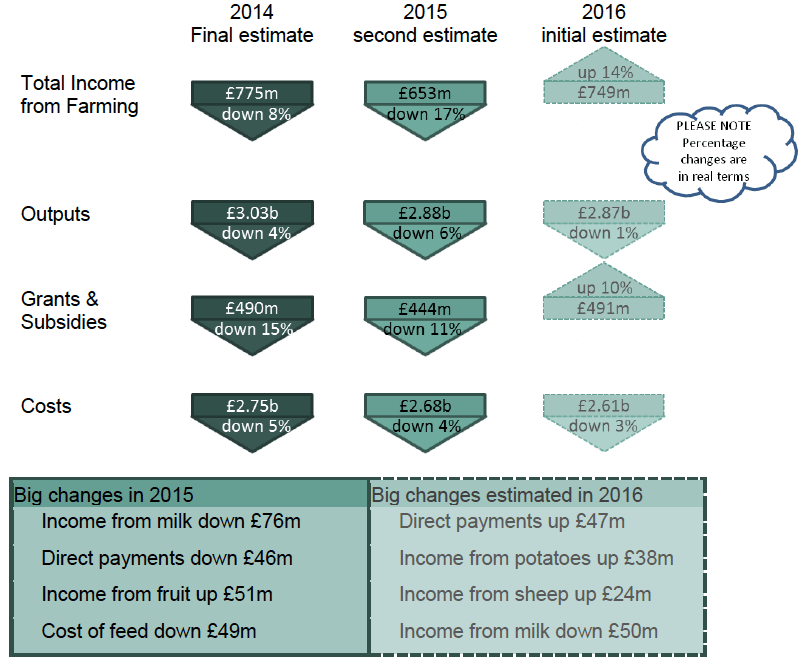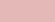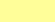Publication - Statistics

# Total income from farming: estimates for Scotland 2014-2016

Published: 31 Jan 2017
Directorate:
Agriculture and Rural Delivery Directorate
Part of:
Farming and rural
ISBN:
9781786527707

Total income from farming (TIFF) is an estimate of the net income from farming in Scotland in the period 2014 to 2016.

4 page PDF

175.1 kB

4 page PDF

175.1 kB

### Supporting files

Contents
Total income from farming: estimates for Scotland 2014-2016
1. Main Findings

4 page PDF

175.1 kB

### 1. Main Findings

The following summary gives estimates of Total Income from Farming for the latest three years, together with their constituent parts, and the percentage changes after accounting for inflation.Why are the results referred to as "estimates"?

This publication contains three sets of estimates of Total Income from Farming ( TIFF)

final estimates for the calendar year 2014
second estimates for the calendar year 2015
initial estimates for the calendar year 2016.

Many of the data used in calculating income from farming for 2016 will only become available during 2017, and some not until 2018. In particular, the final results of the Farm Accounts Survey 2016-17, to be used within many of the 2016 costs estimates, are not available until 2018.

This means that the 2016 TIFF estimates published here contain a large number of forecasts, often based on projecting past trends. In January 2018 we will publish updated "second estimates" for 2016, which may differ substantially from those published here. Final estimates for 2016 will then be published in January 2019.

The tables in this publication give a breakdown of the constituent elements of TIFF. In Table 1 we have attempted to illustrate the degree of certainty in the estimates of each element by means of colour coding.Based heavily on proxy estimatesBased on incomplete dataBased on complete/final data

Why do initial estimates of TIFF often change so much when they are updated?

TIFF is calculated as income minus costs. Income and costs are similarly-sized large figures, giving a relatively small difference as the value of TIFF. This means that a small percentage update in estimates for income or costs may automatically lead to a large percentage revision in TIFF.

For example, if income = 100 and costs = 96, then TIFF = 4. If we then update the estimate for income upwards by just one per cent, and for costs down by just one per cent, income = 101 and costs = 95, so TIFF = 6, a 50 per cent increase in the value of TIFF.

So reasonable small updates in the estimates of income and costs can lead to what may seem an unreasonable 50 per cent change in TIFF. See also note 4.4.

What does "in real terms" mean?

It shows, for previous years, the value of the income or costs expressed in today's prices. This is because, for example, income of £10,000 in 1990 could have paid for something that nowadays costs £20,000, so we say the 1990 income was worth £20,000 in today's prices.

To do this we simply multiply our data on previous years' figures by the amount prices have gone up since then. In our example prices have doubled, so we multiply the 1990 income by two to see what it would be worth in today's prices. For these multipliers we now use the GDP deflator, from the Office for National Statistics ( ONS)  .

### Contact

Email: Neil White